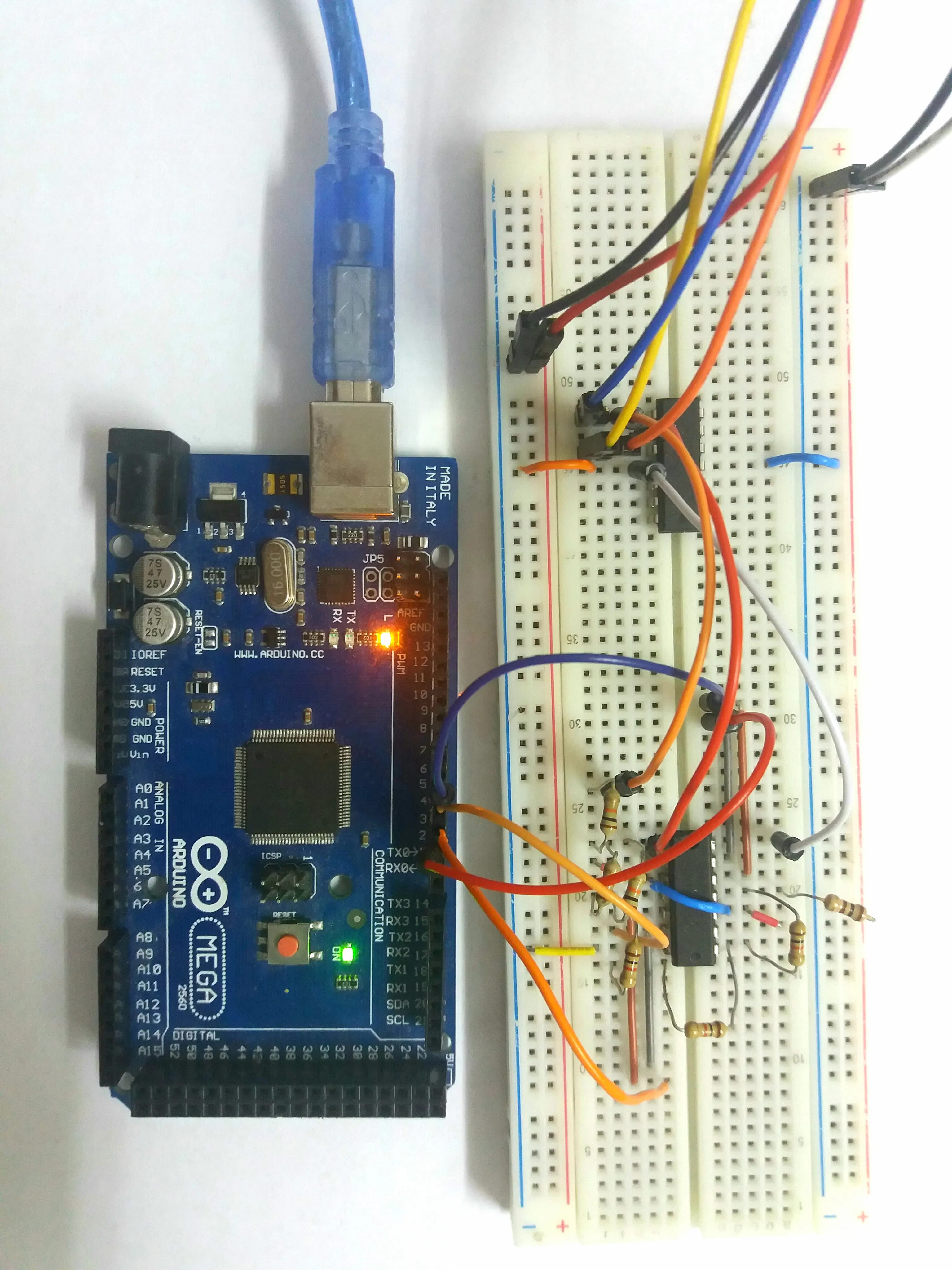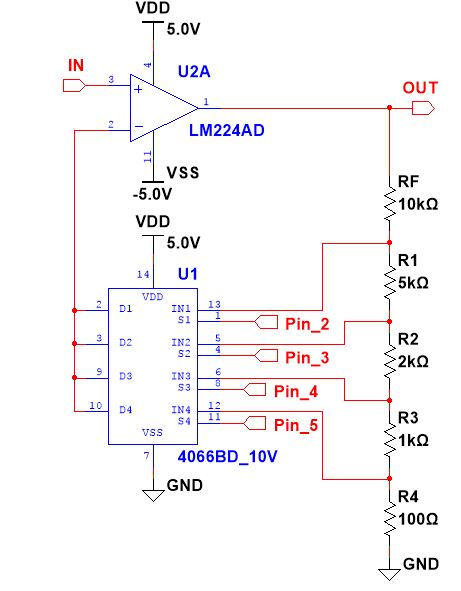Project showcaseArduino Variable Gain Amplifier

Use an Arduino board to control different gain coefficients from an operational amplifier.

• 9,611 views
• 1 comment
• 13 respects

Apps and online services

In signal condition domain, a critical functional block is a VGA or Variable Gain Amplifier. It is used in measurement devices like: the oscilloscope, spectral analyzer, network analyzer and so on.

A simple VGA consists of a few resistors with different values, an operational amplifier and components that switch between these resistors. It can be a solenoid switch or an IC electronic switch. In this case, a SN74HC4066 is a suitable device. It have 4 switches with individual control. A good feature for use with the Arduino board.

So, by making a big voltage divider and choosing between nodes, we can modify the gain equation.

Gain Coefficient

RF stands for resistor feedback. If S1 is close and the rest are open according to OP, the operation gain coefficient will be:

G1 = 1 + RF/(R1+R2+R3+R4)

For S2 closed and the rest open:

G2 = 1 +(RF+R1)/(R2+R3+R4)

And so on:

G3 = 1 +(RF+R1+R2)/(R3+R4)
G4 = 1 +(RF+R1+R2+R3)/(R4)

Therefore, we can obtain 4 gain coefficients. But you may wonder if we can make combinations between gain in order to achieve other equations. Yes, we can and there is more detailed information are here.

So, with this concept in mind, we can make connections between the Arduino board, resistors, IC switch and OP AMP like in the bottom schematic. Pins 2/3/4/5 are connected to Arduino digital pin 2/3/4/5.

Arduino code is very easy because it contains digitalWrite commands and a serial communication to modify gain from the serial monitor.

Code

vga.inoC/C++
void setup() {
pinMode(2, OUTPUT);// to pin 1C
pinMode(3, OUTPUT);// to pin 2C
pinMode(4, OUTPUT);// to pin 3C
pinMode(5, OUTPUT);// to pin 4C
Serial.begin(9600);
}

char cmd;

void loop() {
while (Serial.available() > 0) {

if (cmd == '0') {
digitalWrite(2, 1);
digitalWrite(3, 0);
digitalWrite(4, 0);
digitalWrite(5, 0);
}
if (cmd == '1') {
digitalWrite(2, 0);
digitalWrite(3, 1);
digitalWrite(4, 0);
digitalWrite(5, 0);
}
if (cmd == '2') {
digitalWrite(2, 0);
digitalWrite(3, 0);
digitalWrite(4, 1);
digitalWrite(5, 0);
}
if (cmd == '3') {
digitalWrite(2, 0);
digitalWrite(3, 0);
digitalWrite(4, 0);
digitalWrite(5, 1);
}
if (cmd == '4') {
digitalWrite(2, 1);
digitalWrite(3, 0);
digitalWrite(4, 0);
digitalWrite(5, 1);
}
if (cmd == '5') {
digitalWrite(2, 1);
digitalWrite(3, 0);
digitalWrite(4, 1);
digitalWrite(5, 0);
}
if (cmd == '6') {
digitalWrite(2, 1);
digitalWrite(3, 1);
digitalWrite(4, 0);
digitalWrite(5, 0);
}
if (cmd == '7') {
digitalWrite(2, 0);
digitalWrite(3, 1);
digitalWrite(4, 0);
digitalWrite(5, 1);
}
if (cmd == '8') {
digitalWrite(2, 0);
digitalWrite(3, 1);
digitalWrite(4, 1);
digitalWrite(5, 0);
}
if (cmd == '9') {
digitalWrite(2, 0);
digitalWrite(3, 0);
digitalWrite(4, 1);
digitalWrite(5, 1);
}
}
}

SchematicsArduino Ohm Meter

Project showcase by lucian_vdo

• 12,909 views
• 19 respects

Arduino Mini Shields Construction

Project tutorial by Patel Darshil

• 3,394 views
• 25 respects

Detecting Heart Rate with a Photoresistor

Project tutorial by 3 developers

• 22,940 views
• 46 respects

Arduino Project: Burglar Zone Input Tester

Project tutorial by Cezarjar

• 4,007 views
• 9 respects

Boom Box

Project in progress by Team Trouble

• 5,084 views